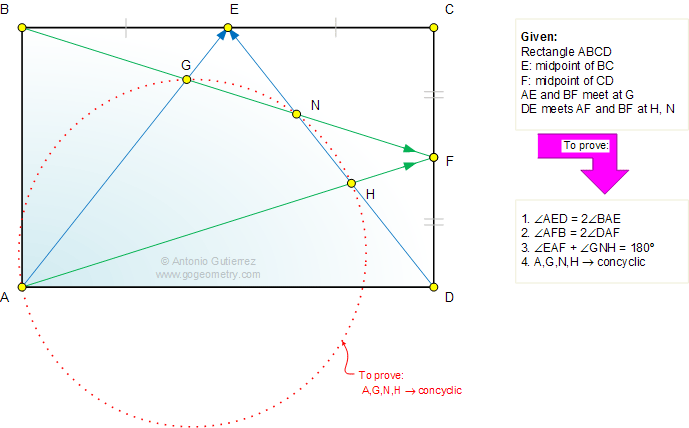# Online Geometry Problem 711: Rectangle, Midpoint, Angle, Concyclic Points, Cyclic Quadrilateral. Level: High School, Honors Geometry, College, Mathematics Education

 The figure shows a rectangle ABCD, E midpoint of BC and F midpoint of CD. AE and BF meet at G. DE meets AF, BF at H, N. Prove that (1) angle AED = 2 angle BAE; (2) angle AFB = 2 angle DAF; (3) angle EAF +  angle GNH = 180 degrees; (4) Points A,G,N,H are concyclic.Home | Search | Geometry | Problems | All Problems | Open Problems | Visual Index | 711-720 | Triangles | Midpoints | Rectangle | Cyclic Quadrilateral | Email | Post a comment or solution | By Antonio Gutierrez